# Domain and Range

When working with functions, we frequently come across two terms: domain & range. What is a domain? What is a range? Why are they important? How can we determine the domain and range for a given function?

#### Definition of Domain

Domain: The set of all possible input values (commonly the “x” variable), which produce a valid output from a particular function. It is the set of all values for which a function is mathematically defined. It is quite common for the domain to be the set of all real numbers since many mathematical functions can accept any input.

For example, many simplistic algebraic functions have domains that may seem… obvious. For the function $$f(x)=2x+1$$, what’s the domain? What values can we put in for the input (x) of this function? Well, anything! The answer is all real numbers. Only when we get to certain types of algebraic expressions will we need to limit the domain.

We can demonstrate the domain visually, as well. Consider a simple linear equation like the graph shown, below drawn from the function $$y=\frac{x}{2}+10$$. What values are valid inputs? It’s not a trick question – every real number is a possible input! The function’s domain is all real numbers because there is nothing you can put in for x that won’t work. Visually we see that as a line that extends forever in the x directions (left and right).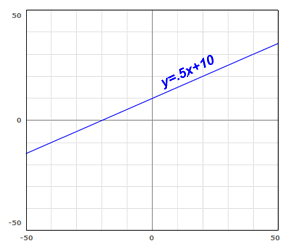For other linear functions (lines), the line might be very, very steep, but if you imagine “zooming out” far enough, eventually any x-value will show up on the graph. A straight, horizontal line, on the other hand, would be the clearest example of an unlimited domain of all real numbers.

What kind of functions don’t have a domain of all real numbers? What would stop us, as algebra students, from inserting any value into the input of a function? Well, if the domain is the set of all inputs for which the function is defined, then logically we’re looking for an example function which breaks for certain input values. We need a function that, for certain inputs, does not produce a valid output, i.e., the function is undefined for that input. Here is an example:

This function is defined for almost any real x. But, what is the value of y when x=1? Well, it’s $$\frac{3}{0}$$, which is undefined. Division by zero is undefined. Therefore 1 is not in the domain of this function. We cannot use 1 as an input, because it breaks the function. All other real numbers are valid inputs, so the domain is all real numbers except for x=1. Makes sense, right?

Division by zero is one of the very most common places to look when solving for a function’s domain. Look for places that could result in a division by zero condition, and write down the x-values that cause the denominator to be zero. Those are your values to exclude from the domain.

If division by zero is a common place to look for limits on the domain, then the “square root” sign is probably the second-most common. Of course, we know it’s really called the radical symbol, but undoubtedly you call it the square root sign. Why does that cause issues with the domain? Because, at least in the realm of real numbers, we cannot solve for the square root of a negative value.

What if we’re asked to find the domain of $$f(x)=\sqrt{x-2}$$. What values are excluded from the domain? Anything less than 2 results in a negative number inside the square root, which is a problem. Therefore the domain is all real numbers greater than or equal to 2.

What other kinds of functions have domains that aren’t all real numbers? Certain “inverse” functions, like the inverse trig functions, have limited domains as well. Since the sine function can only have outputs from -1 to +1, its inverse can only accept inputs from -1 to +1. The domain of inverse sine is -1 to +1. However, the most common example of a limited domain is probably the divide by zero issue. When asked to find the domain of a function, start with the easy stuff: first look for any values that cause you to divide by zero. Remember also that we cannot take the square root of a negative number, so keep an eye out for situations where the radicand (the “stuff” inside the square root sign) could result in a negative value. In that case, it would not be a valid input so the domain would not include such values.

#### Definition of Range

Range: The range is the set of all possible output values (commonly the variable y, or sometimes expressed as $$f(x)$$), which result from using a particular function.

The range of a simple, linear function is almost always going to be all real numbers. A graph of a typical line, such as the one shown below, will extend forever in either y direction (up or down). The range of a non-horizontal linear function is all real numbers no matter how flat the slope might look.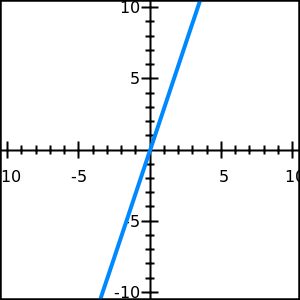There's one notable exception: when y equals a constant (like $$y=4$$ or $$y=19$$). When you have a function where y equals a constant, your graph is a truly horizontal line, like the graph below of $$y=3$$. In that case, the range is just that one and only value. No other possible values can come out of that function!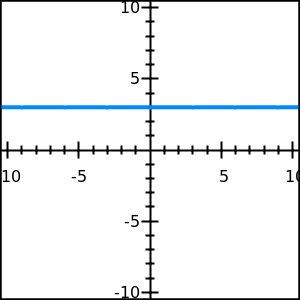Many other functions have limited ranges. While only a few types have limited domains, you will frequently see functions with unusual ranges. Here are a few examples below. The blue line represents $$y=x^2-2$$, while the red curve represents $$y=\sin{x}$$.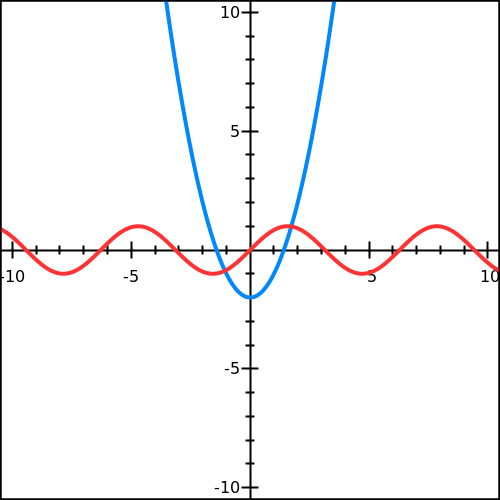As you can see, these two functions have ranges that are limited. No matter what values you enter into a sine function you will never get a result greater than 1 or less than -1. No matter what values you enter into $$y=x^2-2$$ you will never get a result less than -2.

How can we identify a range that isn't all real numbers? Like the domain, we have two choices. We can look at the graph visually (like the sine wave above) and see what the function is doing, then determine the range, or we can consider it from an algebraic point of view. Variables raised to an even power ($$x^2$$, $$x^4$$, etc...) will result in only positive output, for example. Special-purpose functions, like trigonometric functions, will also certainly have limited outputs.

Summary: The domain of a function is all the possible input values for which the function is defined, and the range is all possible output values.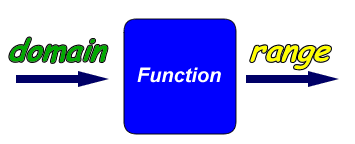If you are still confused, you might consider posting your question on our message board, or reading another website's lesson on domain and range to get another point of view. Or, you can use the calculator below to determine the domain and range of ANY equation: